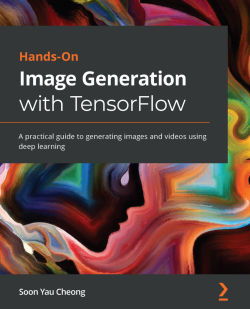Hands-On Image Generation with TensorFlow

By Soon Yau Cheong
• \$5/mo for 5 months Subscribe Access now
• \$49.99 Print + eBook
• \$34.99 eBook

• Constantly updated with 100+ new titles each month
• Breadth and depth in over 1,000+ technologies

The emerging field of Generative Adversarial Networks (GANs) has made it possible to generate indistinguishable images from existing datasets. With this hands-on book, you’ll not only develop image generation skills but also gain a solid understanding of the underlying principles.

Starting with an introduction to the fundamentals of image generation using TensorFlow, this book covers Variational Autoencoders (VAEs) and GANs. You’ll discover how to build models for different applications as you get to grips with performing face swaps using deepfakes, neural style transfer, image-to-image translation, turning simple images into photorealistic images, and much more. You’ll also understand how and why to construct state-of-the-art deep neural networks using advanced techniques such as spectral normalization and self-attention layer before working with advanced models for face generation and editing. You'll also be introduced to photo restoration, text-to-image synthesis, video retargeting, and neural rendering. Throughout the book, you’ll learn to implement models from scratch in TensorFlow 2.x, including PixelCNN, VAE, DCGAN, WGAN, pix2pix, CycleGAN, StyleGAN, GauGAN, and BigGAN.

By the end of this book, you'll be well versed in TensorFlow and be able to implement image generative technologies confidently.

Publication date:
December 2020
Publisher
Packt
Pages
306
ISBN
9781838826789

Chapter 1: Getting Started with Image Generation Using TensorFlow

This book focuses on generating images and videos using unsupervised learning with TensorFlow 2. We assume that you have prior experience in using modern machine learning frameworks, such as TensorFlow 1, to build image classifiers with Convolutional Neural Networks (CNNs). Therefore, we will not be covering the basics of deep learning and CNNs. In this book, we will mainly use high level Keras APIs in TensorFlow 2, which is easy to learn. Nevertheless, we assume that you have no prior knowledge of image generation, and we will go through all that is needed to help you get started with it. The first aspect that you need to know about is probability distribution.

Probability distribution is fundamental in machine learning and it is especially important in generative models. Don't worry, I assure you that there aren't any complex mathematical equations in this chapter. We will first learn what probability is and how to use it to generate faces without using any neural networks or complex algorithms.

That's right: with the help of only basic math and NumPy code, you'll learn how to create a probabilistic generative model. Following that, you will learn how to use TensorFlow 2 to build a PixelCNN model in order to generate handwritten digits. This chapter is packed with useful information; you will need to read this chapter before jumping to any other chapters.

In this chapter, we are going to cover the following main topics:

• Understanding probabilities
• Generating faces with a probabilistic model
• Building a PixelCNN model from scratch

Understanding probabilities

You can't escape the term probability in any machine learning literature, and it can be confusing as it can have different meanings in different contexts. Probability is often denoted as p in mathematical equations, and you see it everywhere in academic papers, tutorials, and blogs. Although it is a concept that is seemingly easy to understand, it can be quite confusing. This is because there are multiple different definitions and interpretations depending on the context. We will use some examples to clarify things. In this section, we will go over the use of probability in the following contexts:

• Distribution
• Belief

Probability distribution

Say we want to train a neural network to classify images of cats and dogs and that we found a dataset that contains 600 images of dogs and 400 images of cats. As you may be aware, the data will need to be shuffled before being fed into the neural network. Otherwise, if it sees only images of the same label in a minibatch, the network will get lazy and say all images have the same label without taking the effort to look hard and differentiate between them. If we sampled the dataset randomly, the probabilities could be written as follows:

pdata(dog) = 0.6

pdata(cat) = 0.4

The probabilities here refer to the data distribution. In this example, this refers to the ratio of the number of cat and dog images to the total number of images in the dataset. The probability here is static and will not change for a given dataset.

When training a deep neural network, the dataset is usually too big to fit into one batch, and we need to break it into multiple minibatches for one epoch. If the dataset is well shuffled, then the sampling distribution of the minibatches will resemble that of the data distribution. If the dataset is unbalanced, where some classes have a lot more images from one label than another, then the neural network may be biased toward predicting the images it sees more. This is a form of overfitting. We can therefore sample the data differently to give more weight to the less-represented classes. If we want to balance the classes in sampling, then the sampling probability becomes as follows:

psample(dog) = 0.5

psample(cat) = 0.5

Note

Probability distribution p(x) is the probability of the occurrence of a data point x. There are two common distributions that are used in machine learning. Uniform distribution is where every data point has the same chances of occurrence; this is what people normally imply when they say random sampling without specifying the distribution type. Gaussian distribution is another commonly used distribution. It is so common that people also call it normal distribution. The probabilities peak at the center (mean) and slowly decay on each side. Gaussian distribution also has nice mathematical properties that make it a favorite of mathematicians. We will see more of that in the next chapter.

Prediction confidence

After several hundred iterations, the model has finally finished training, and I can't wait to test the new model with an image. The model outputs the following probabilities:

p(dog) = 0.6

p(cat) = 0.4

Wait, is the AI telling me that this animal is a mixed-breed with 60% dog genes and 40% cat inheritance? Of course not!

Here, the probabilities no longer refer to distributions; instead, they tell us how confident we can be about the predictions, or in other words, how strongly we can believe in the output. Now, this is no longer something you quantify by counting occurrences. If you are absolutely sure that something is a dog, you can put p(dog) = 1.0 and p(cat) = 0.0. This is known as Bayesian probability.

Note

The traditional statistics approach sees probability as the chances of the occurrence of an event, for example, the chances of a baby being a certain sex. There has been great debate in the wider statistical field on whether the frequentist or Bayesian method is better, which is beyond the scope of this book. However, the Bayesian method is probably more important in deep learning and engineering. It has been used to develop many important algorithms, including Kalman filtering to track rocket trajectory. When calculating the projection of a rocket's trajectory, the Kalman filter uses information from both the global positioning system (GPS) and speed sensor. Both sets of data are noisy, but GPS data is less reliable initially (meaning less confidence), and hence this data is given less weight in the calculation. We don't need to learn the Bayesian theorem in this book; it's enough to understand that probability can be viewed as a confidence score rather than as frequency. Bayesian probability has also recently been used in searching for hyperparameters for deep neural networks.

We have now clarified two main types of probabilities commonly used in general machine learning – distribution and confidence. From now on, we will assume that probability means probability distribution rather than confidence. Next, we will look at a distribution that plays an exceptionally important role in image generation – pixel distribution.

The joint probability of pixels

Take a look at the following pictures – can you tell whether they are of dogs or cats? How do you think the classifier will produce the confidence score?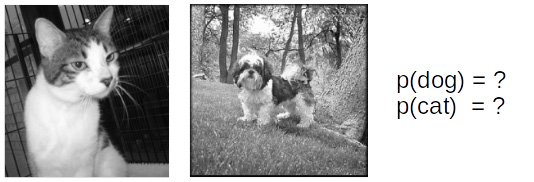Figure 1.1 – Pictures of a cat and a dog

Are either of these pictures of dogs or cats? Well, the answer is pretty obvious, but at the same time it's not important to what we are going to talk about. When you looked at the pictures, you probably thought in your mind that the first picture was of a cat and the second picture was of a dog. We see the picture as a whole, but that is not what the computer sees. The computer sees pixels.

Note

A pixel is the smallest spatial unit in digital image, and it represents a single color. You cannot have a pixel where half is black and the other half is white. The most commonly used color scheme is 8-bit RGB, where a pixel is made up of three channels named R (red), G (green), and B (blue). Their values range from 0 to 255 (255 being the highest intensity). For example, a black pixel has a value of [0, 0, 0], while a white pixel is [255, 255, 255].

The simplest way to describe the pixel distribution of an image is by counting the number of pixels that have different intensity levels from 0 to 255; you can visualize this by plotting a histogram. It is a common tool in digital photography to look at a histogram of separate R, G, and B channels to understand the color balance. Although this can provide some information to us – for example, an image of sky is likely to have many blue pixels, so a histogram may reliably tell us something about that – histograms do not tell us how pixels relate to each other. In other words, a histogram does not contain spatial information, that is, how far a blue pixel is from another blue pixel. We will need a better measure for this kind of thing.

Instead of saying p(x), where x is a whole image, we can define x as x1, x2, x3,… xn. Now, p(x) can be defined as the joint probability of pixels p(x1, x2, x3,… xn), where n is the number of pixels and each pixel is separated by a comma.

We will use the following images to illustrate what we mean by joint probability. The following are three images with 2 x 2 pixels that contain binary values, where 0 is black and 1 is white. We will call the top-left pixel x1, the top-right pixel x2, the bottom-left pixel x3, and the bottom-right pixel x4: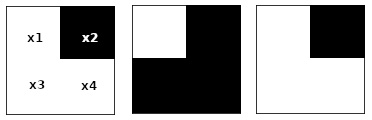Figure 1.2 – Images with 2 x 2 pixels

We first calculate p(x1 = white) by counting the number of white x1 and dividing it by the total number of the image. Then, we do the same for x2, as follows:

p(x1 = white)  = 2 / 3

p(x2 = white)  = 0 / 3

Now we say that p(x1) and p(x2) are independent of each other because we calculated them separately. If we calculate the joint probability where both x1 and x2 are black, we get the following:

p(x1 = black, x2 = black)  = 0 / 3

We can then calculate the complete joint probability of these two pixels as follows:

p(x1 = black, x2 = white)  = 0 / 3

p(x1 = white, x2 = black)  = 3 / 3

p(x1 = white, x2 = white)  = 0 / 3

We'll need to do the same steps 16 times to calculate the complete joint probability of p(x1, x2, x3, x4). Now, we could fully describe the pixel distribution and use that to calculate the marginal distribution, as in p(x1, x2, x3) or p(x1). However, the calculations required for the joint distribution increase exponentially for RGB values where each pixel has 256 x 256 x 256  = 16,777,216 possibilities. This is where deep neural networks come to the rescue. A neural network can be trained to learn a pixel data distribution Pdata. Hence, a neural network is our probability model Pmodel.

Important Note

The notations we will use in this book are as follows: capital X for the dataset, lowercase x for image sampled from the dataset, and lowercase with subscript xi for the pixel.

The purpose of image generation is to generate an image that has a pixel distribution p(x) that resembles p(X). For example, an image dataset of oranges will have a high probability of lots of occurrences of orange pixels that are distributed close to each other in a circle. Therefore, before generating image, we will first build a probability model pmodel(x) from real data pdata(X). After that, we generate images by drawing a sample from pmodel(x).

Generating faces with a probabilistic model

Alright, enough mathematics. It is now time to get your hands dirty and generate your first image. In this section, we will learn how to generate images by sampling from a probabilistic model without even using a neural network.

Mean faces

We will be using the large-scale CelebFaces Attributes (CelebA) dataset created by The Chinese University of Hong Kong (http://mmlab.ie.cuhk.edu.hk/projects/CelebA.html). This can be downloaded directly with Python's tensorflow_datasets module within the ch1_generate_first_image.ipynb Jupyter notebook, as shown in the following code:

import tensorflow_datasets as tfds
import matplotlib.pyplot as plt
import numpy as np
shuffle_files=False,
with_info=True)
fig = tfds.show_examples(ds_info, ds_train)

The TensorFlow dataset allows us to preview some examples of images by using the tfds.show_examples() API. The following are some samples of male and female celebrities' faces: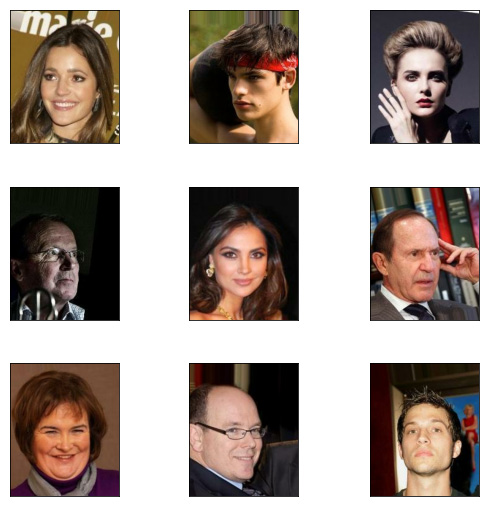Figure 1.3 – Sample images from the CelebA dataset

As you can see in the figure, there is a celebrity face in every image. Every picture is unique, with a variety of genders, poses, expressions, and hairstyles; some wear glasses and some don't. Let's see how to exploit the probability distribution of the images to help us create a new face. We'll use one of the simplest statistical methods – the mean, which means taking an average of the pixels from the images. To be more specific, we are averaging the xi of every image to calculate the xi of a new image. To speed up the processing, we'll use only 2,000 samples from the dataset for this task, as follows:

sample_size = 2000
ds_train = ds_train.batch(sample_size)
features = next(iter(ds_train.take(1)))
sample_images = features['image']
new_image = np.mean(sample_images, axis=0)
plt.imshow(new_image.astype(np.uint8))

Ta-dah! That is your first generated image, and it looks pretty amazing! I initially thought it would look a bit like one of Picasso's paintings, but it turns out that the mean image is quite coherent: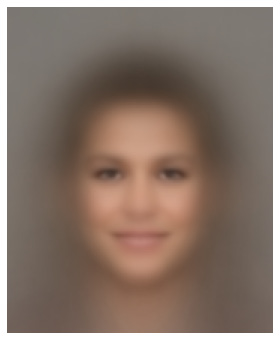Figure 1.4 – The mean face

Conditional probability

The best thing about the CelebA dataset is that each image is labeled with facial attributes as follows: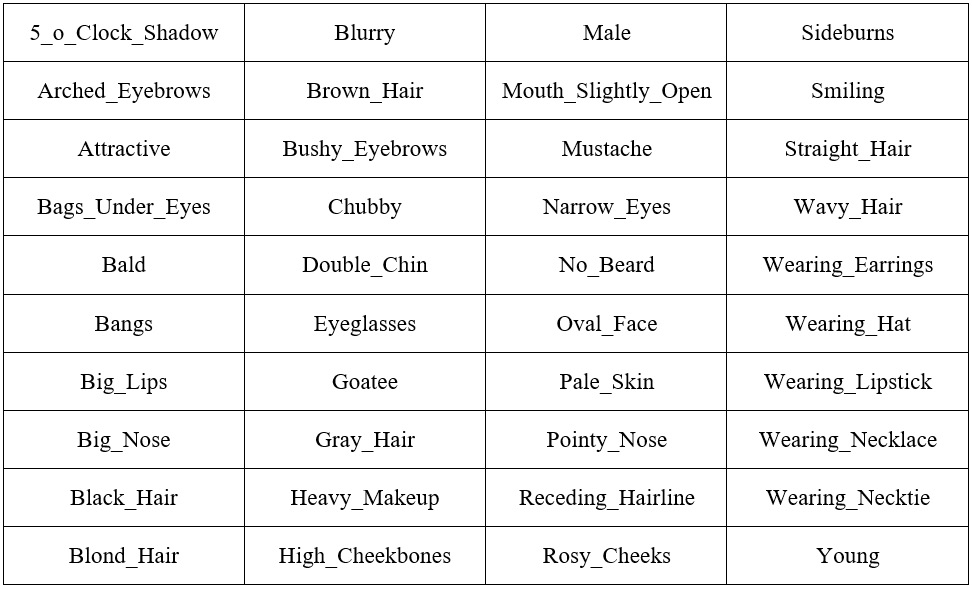Figure 1.5 – 40 attributes in the CelebA dataset in alphabetical order

We are going to use these attributes to generate a new image. Let's say we want to generate a male image. How do we do that? Instead of calculating the probability of every image, we use only images that have the Male attribute set to true. We can put it in this way:

p(x | y)

We call this the probability of x conditioned on y, or more informally the probability of x given y. This is called conditional probability. In our example, y is the facial attributes. When we condition on the Male attribute, this variable is no longer a random probability; every sample will have the Male attribute and we can be certain that every face belongs to a man. The following figure shows new mean faces generated using other attributes as well as Male, such as Male + Eyeglasses and Male + Eyeglasses + Mustache + Smiling. Notice that as the conditions increase, the number of samples reduces and the mean image also becomes noisier: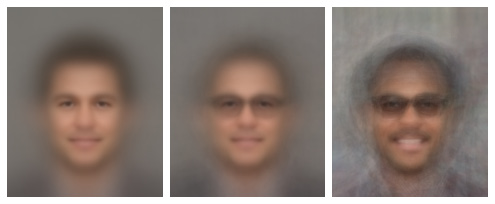Figure 1.6 – Adding attributes from left to right. (a) Male (b) Male + Eyeglasses (c) Male + Eyeglasses + Mustache + Smiling

You could use the Jupyter notebook to generate a new face by using different attributes, but not every combination produces satisfactory results. The following are some female faces generated with different attributes. The rightmost image is an interesting one. I used attributes of Female, Smiling, Eyeglasses, and Pointy_Nose, but it turns out that people with these attributes tend to also have wavy hair, which is an attribute that was excluded in this sample. Visualization can be a useful tool to provide insights into your dataset: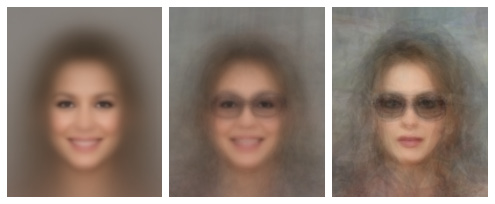Figure 1.7 – Female faces with different attributes

Tips

Instead of using the mean when generating images, you can try to using the median as well, which may produce a sharper image. Simply replace np.mean() with np.median().

Probabilistic generative models

There are three main goals that we wish to achieve with image-generation algorithms:

1. Generate images that look like ones in the given dataset.
2. Generate a variety of images.
3. Control the images being generated.

By simply taking the mean of the pixels in an image, we have demonstrated how to achieve goals 1 and 3. However, one limitation is that we could only generate one image per condition. That really isn't very effective for an algorithm, generating only one image from hundreds or thousands of training images.

The following chart shows the distribution of one color channel of an arbitrary pixel in the dataset. The x mark on the chart is the median value. When we use the mean or median of data, we are always sampling the same point, and therefore there is no variation in the outcome. Is there a way to generate multiple different faces? Yes, we can try to increase the generated image variation by sampling from the entire pixel distribution: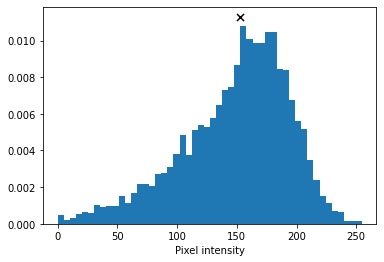Figure 1.8 – The distribution of a pixel's color channel

A machine learning textbook will probably ask you to first create a probabilistic model, pmodel, by calculating the joint probability of every single pixel. But as the sample space is huge (remember, one RGB pixel can have 16,777,216 different values), it is computationally expensive to implement. Also, because this is a hands-on book, we will draw pixel samples directly from datasets. To create an x0 pixel in a new image, we randomly sample from an x0 pixel of all images in the dataset by running the following code:

new_image = np.zeros(sample_images.shape[1:], dtype=np.uint8)
for i in range(h):
for j in range(w):
rand_int = np.random.randint(0, sample_images.shape)
new_image[i,j] = sample_images[rand_int,i,j]

Images were generated using random sampling. Disappointingly, although there is some variation between the images, they are not that different from each other, and one of our objectives is to be able to generate a variety of faces. Also, the images are noticeably noisier than when using the mean. The reason for this is that the pixel distribution is independent of each other.

For example, for a given pixel in the lips, we can reasonably expect the color to be pink or red, and the same goes for the adjacent pixels. Nevertheless, because we are sampling independently from images where faces appear in different locations and poses, this results in color discontinuities between pixels, ultimately giving this noisy result: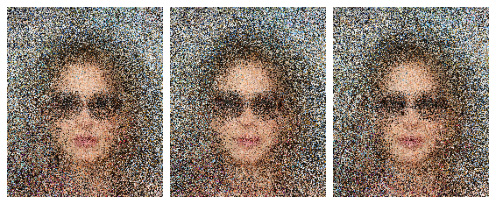Figure 1.9 – Images generated by random sampling

Tips

You may be wondering why the mean face looks smoother than with random sampling. Firstly, it is because the distance of the mean between pixels is smaller. Imagine a random sampling scenario where one pixel sampled is close to 0 and the next one is close to 255. The mean of these pixels would likely lie somewhere in the middle, and therefore the difference between them would be smaller. On the other hand, pixels in the backgrounds of pictures tend to have a uniform distribution; for example, they could all be part of a blue sky, a white wall, green leaves, and so on. As they are distributed rather evenly across the color spectrum, the mean value is around [127, 127, 127], which happens to be gray.

Parametric modeling

What we just did was use a pixel histogram as our pmodel, but there are a few shortcomings here. Firstly, due to the large sample space, not every possible color exists in our sample distribution. As a result, the generated image will never contain any colors that are not present in the dataset. For instance, we want to be able to generate the full spectrum of skin tones rather than only one very specific shade of brown that exists in the dataset. If you did try to generate faces using conditions, you will have found that not every combination of conditions is possible. For example, for Mustache + Sideburns + Heavy_Makeup + Wavy_Hair, there simply wasn't a sample that met those conditions!

Secondly, the sample spaces increase as we increase the size of the dataset or the image resolution. This can be solved by having a parameterized model. The vertical bar chart in the following figure shows a histogram of 1,000 randomly generated numbers: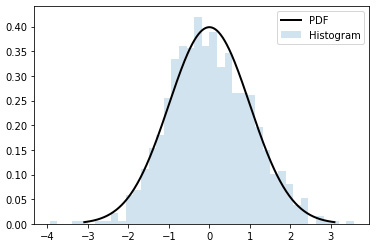Figure 1.10 – Gaussian histogram and model

We can see that there are some bars that don't have any value. We can fit a Gaussian model on the data in which the Probability Density Function (PDF) is plotted as a black line. The PDF equation for a Gaussian distribution is as follows: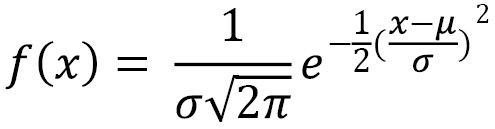Here, µ is the mean and σ is the standard deviation.

We can see that the PDF covers the histogram gap, which means we can generate a probability for the missing numbers. This Gaussian model has only two parameters – the mean and the standard variation.

The 1,000 numbers can now be condensed to just two parameters, and we can use this model to draw as many samples as we wish; we are no longer limited to the data we fit the model with. Of course, natural images are complex and could not be described by simple models such as a Gaussian model, or in fact any mathematical models. This is where neural networks come into play. Now we will use a neural network as a parameterized image-generation model where the parameters are the network's weights and biases.

Building a PixelCNN model from scratch

There are three main categories of deep neural network generative algorithms:

• Variational Autoencoders (VAEs)
• Autoregressive models

VAEs will be introduced in the next chapter, and we will use them in some of our models. The GAN is the main algorithm we will be using in this book, and there are a lot more details about it to come in later chapters. We will introduce the lesser-known autoregressive model family here and focus on VAEs and GANs later in the book. Although it is not so common in image generation, autoregression is still an active area of research, with DeepMind's WaveNet using it to generate realistic audio. In this section, we will introduce autoregressive models and build a PixelCNN model from scratch.

Autoregressive models

Auto here means self, and regress in machine learning terminology means predict new values. Putting them together, autoregressive means we use a model to predict new data points based on the model's past data points.

Let's recall the probability distribution of an image is p(x) is joint pixel probability p(x1, x2,… xn) which is difficult to model due to the high dimensionality. Here, we make an assumption that the value of a pixel depends only on that of the pixel before it. In other words, a pixel is conditioned only on the pixel before it, that is, p(xi) = p(xi | xi-1) p(xi-1). Without going into the mathematical details, we can approximate the joint probability to be the product of conditional probabilities:

p(x) = p(xn, xn-1, …, x2, x1)

p(x) = p(xn | xn-1)... p(x3 | x2) p(x2 | x1) p(x1)

To give a concrete example, let's say we have images that contain only a red apple in roughly the center of the image, and that the apple is surrounded by green leaves. In other words, only two colors are possible: red and green. x1 is the top-left pixel, so p(x1) is the probability of whether the top-left pixel is green or red. If x1 is green, then the pixel to its right p(x2) is likely to be green too, as it's likely to be more leaves. However, it could be red, despite the smaller probability.

As we go on, we will eventually hit a red pixel (hooray! We have found our apple!). From that pixel onward, it is likely that the next few pixels are more likely to be red too. We can now see that this is a lot simpler than having to consider all of the pixels together.

PixelRNN

PixelRNN was invented by the Google-acquired DeepMind back in 2016. As the name RNN (Recurrent Neural Network) suggests, this model uses a type of RNN called Long Short-Term Memory (LSTM) to learn an image's distribution. It reads the image one row at a time in a step in the LSTM and processes it with a 1D convolution layer, then feeds the activations into subsequent layers to predict pixels for that row.

As LSTM is slow to run, it takes a long time to train and generate samples. As a result, it fell out of fashion and there has not been much improvement made to it since its inception. Thus, we will not dwell on it for long and will instead move our attention to a variant, PixelCNN, which was also unveiled in the same paper.

Building a PixelCNN model with TensorFlow 2

PixelCNN is made up only of convolutional layers, making it a lot faster than PixelRNN. Here, we will implement a simple PixelCNN model for MNIST. The code can be found in ch1_pixelcnn.ipynb.

Input and label

MNIST consists of 28 x 28 x 1 grayscale images of handwritten digits. It only has one channel, with 256 levels to depict the shade of gray: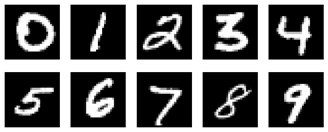Figure 1.11 – MNIST digit examples

In this experiment, we simplify the problem by casting images into binary format with only two possible values: 0 represents black and 1 represents white. The code for this is as follows:

def binarize(image, label):
image = tf.cast(image, tf.float32)
image = tf.math.round(image/255.)
return image, tf.cast(image, tf.int32)

The function expects two inputs – an image and a label. The first two lines of the function cast the image into binary  float32 format, in other words 0.0 or 1.0. In this tutorial, we will not use the label information; instead, we cast the binary image into an integer and return it. We don't have to cast it to an integer, but let's just do it to stick to the convention of using an integer for labels. To recap, both the input and the label are binary MNIST images of 28 x 28 x 1; they differ only in data type.

Unlike PixelRNN, which reads row by row, PixelCNN slides a convolutional kernel across the image from left to right and from top to bottom. When performing convolution to predict the current pixel, a conventional convolution kernel is able to see the current input pixel together with the pixels surrounding it, including future pixels, and this breaks our conditional probability assumptions.

To avoid that, we need to make sure that the CNN doesn't cheat to look at the pixel it is predicting. In other words, we need to make sure that the CNN doesn't see the input pixel xi while it is predicting the output pixel xi.

This is by using a masked convolution, where a mask is applied to the convolutional kernel weights before performing convolution. The following diagram shows a mask for a 7 x 7 kernel, where the weight from the center onward is 0. This blocks the CNN from seeing the pixel it is predicting (the center of the kernel) and all future pixels. This is known as a type A mask and is applied only to the input layer. As the center pixel is blocked in the first layer, we don't need to hide the center feature anymore in later layers. In fact, we will need to set the kernel center to 1 to enable it to read the features from previous layers. This is known as type B Mask: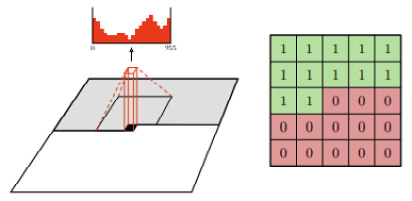Figure 1.12 – A 7 x 7 kernel mask (Source: Aäron van den Oord et al., 2016,  Conditional Image Generation with PixelCNN Decoders, https://arxiv.org/abs/1606.05328)

Next, we will learn how to create a custom layer.

Implementing a custom layer

We will now create a custom layer for the masked convolution. We can create a custom layer in TensorFlow using model subclassing inherited from the base class, tf.keras.layers.Layer, as shown in the following code. We will be able to use it just like other Keras layers. The following is the basic structure of the custom layer class:

def __init__(self):
...
def build(self, input_shape):
...
def call(self, inputs):
...
return output

build() takes the input tensor's shape as an argument, and we will use this information to create variables of the correct shapes. This function runs only once, when the layer is built. We can create a mask by declaring it either as a non-trainable variable or as a constant to let TensorFlow know it does not need to have gradients to backpropagate:

def build(self, input_shape):
self.kernel,
input_shape[-1],
self.filters],
initializer='glorot_normal',
trainable=True)
initializer='zeros',
trainable=True)

call() is the forward pass that performs the computation. In this masked convolutional layer, we multiply the weight by the mask to zero the lower half before performing convolution using the low-level tf.nn API:

def call(self, inputs):
output = tf.nn.conv2d(inputs, masked_w, 1, "SAME") +  	                   self.b
return output

Tips

tf.keras.layers is a high-level API that is easy to use without you needing to know under-the-hood details. However, sometimes we will need to create custom functions using the low-level tf.nn API, which requires us to first specify or create the tensors to be used.

Network layers

The PixelCNN architecture is quite straightforward. After the first 7 x 7 conv2d layer with mask A, there are several layers of residual blocks (see the following table) with mask B. To keep the same feature map size of 28 x 28, there is no downsampling; for example, the max pooling and padding in these layers is set to SAME. The top features are then fed into two layers of 1 x 1 convolution layers before the output is produced, as seen in the following screenshot: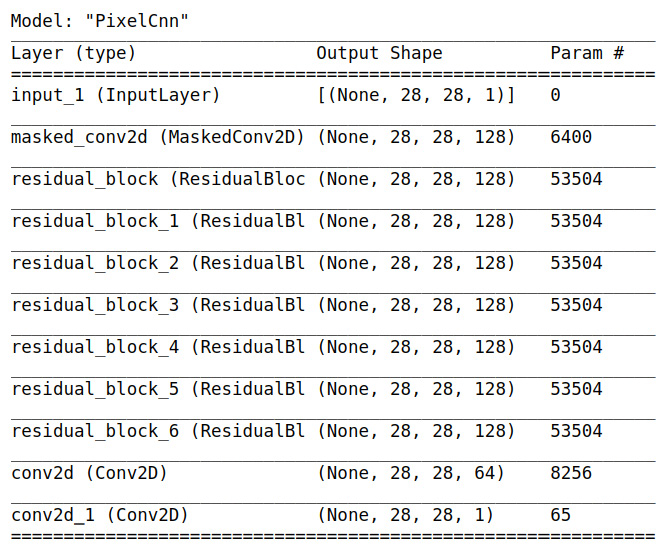Figure 1.13 – The PixelCNN architecture, showing the layers and output shape

Residual blocks are used in many high-performance CNN-based models and were made popular by ResNet, which was invented by Kaiming He et al. in 2015. The following diagram illustrates a variant of residual blocks used in PixelCNN. The left path is called a skip connection path, which simply passes the features from the previous layer. On the right path are three sequential convolutional layers with filters of 1 x 1, 3 x 3, and 1 x 1. This path optimizes the residuals of the input features, hence the name residual net: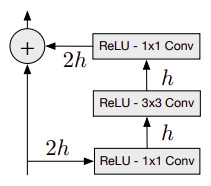Figure 1.14 – The residual block where h is the number of filters. (Source: Aäron van den Oord et al., Pixel Recurrent Neural Networks)

Cross-entropy loss

Cross-entropy loss, also known as log loss, measures the performance of a model, where the output's probability is between 0 and 1. The following is the equation for binary cross-entropy loss, where there are only two classes, labels y can be either 0 or 1, and p(x) is the model's prediction. The equation is as follows: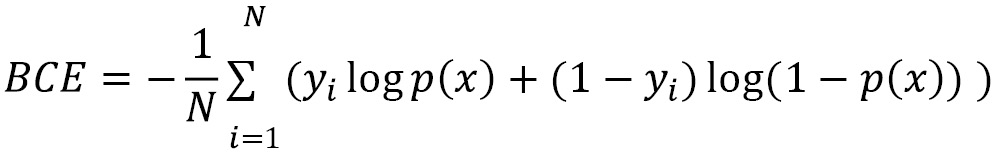Let's look at an example where the label is 1, the second term is zero, and the first term is the sum of log p(x). The log in the equation is natural log (loge) but by convention the base of e is omitted from the equations. If the model is confident that x belongs to label 1, then log(1) is zero. On the other hand, if the model wrongly guesses it as label 0 and predicts a low probability of x being label 1, say p(x) = 0.1. Then -log (p(x)) becomes higher loss of 2.3. Therefore, minimizing cross-entropy loss will maximize the model's accuracy. This loss function is commonly used in classification models but is also popular among generative models.

In PixelCNN, the individual image pixel is used as a label. In our binarized MNIST, we want to predict whether the output pixel is either 0 or 1, which makes this a classification problem with cross-entropy as the loss function.

There can be two output types:

• Since there can only be 0 or 1 in a binarized image, we can simplify the network by using sigmoid() to predict the probability of a white pixel, that is, p(xi = 1). The loss function is binary cross-entropy. This is what we will use in our PixelCNN model.
• Optionally, we could also generalize the network to accept grayscale or RGB images. We can use the softmax() activation function to produce N probabilities for each (sub)pixel. N will be 2 for binarized images, 256 for grayscale images, and 3 x 256 for RGB images. The loss function is sparse categorical cross-entropy or categorical cross-entropy if the label is one-hot encoded.

Finally, we are now ready to compile and train the neural network. As seen in the following code, we use binary cross-entropy for both loss and metrics and use RMSprop as the optimizer. There are many different optimizers to use, and their main difference comes in how they adjust the learning rate of individual variables based on past statistics. Some optimizers accelerate training but may tend to overshoot and not achieve global minima. There is no one best optimizer to use in all cases, and you are encouraged to try different ones.

However, the two optimizers that you will see a lot are Adam and RMSprop. The Adam optimizer is a popular choice in image generation for its fast learning, while RMSprop is used frequently by Google to produce state-of-the-art models.

The following is used to compile and fit the pixelcnn model:

pixelcnn = SimplePixelCnn()
pixelcnn.compile(
loss = tf.keras.losses.BinaryCrossentropy(),
optimizer=tf.keras.optimizers.RMSprop(learning_rate=0.001),
metrics=[ tf.keras.metrics.BinaryCrossentropy()])
pixelcnn.fit(ds_train, epochs = 10, validation_data=ds_test)

Next, we will generate a new image from the preceding model.

Sample image

After the training, we can generate a new image using the model by taking the following steps:

1. Create an empty tensor with the same shape as the input image and fill it with zeros. Feed this into the network and get p(x1), the probability of the first pixel.
2. Sample from p(x1) and assign the sample value to pixel x1 in the input tensor.
3. Feed the input to the network again and perform step 2 for the next pixel.
4. Repeat steps 2 and 3 until xN has been generated.

One major drawback of the autoregressive model is that it is slow because of the need to generate pixel by pixel, which cannot be parallelized. The following images were generated by our simple PixelCNN model after 50 epochs of training. They don't look quite like proper digits yet, but they're starting to take the shape of handwriting strokes. It's quite amazing that we can now generate new images out of thin air (that is, with zero-input tensors). Can you generate better digits by training the model longer and doing some hyperparameter tuning?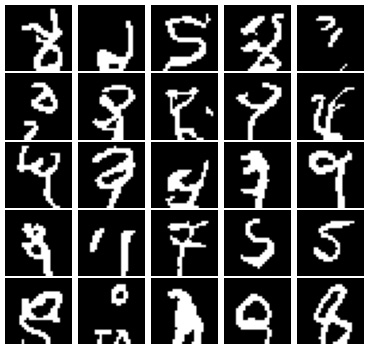Figure 1.15 – Some images generated by our PixelCNN model

With that, we have come to the end of the chapter!

Summary

Wow! I think we have learned a lot in this first chapter, from understanding pixel probability distribution to using it to build a probabilistic model to generate images. We learned how to build custom layers with TensorFlow 2 and use them to construct autoregressive PixelCNN models to generate images of handwritten digits.

In the next chapter, we will learn how to do representation with VAEs. This time, we will look at pixels from a whole new perspective. We will train a neural network to learn facial attributes, and you'll perform face edits, such as morphing a sad-looking girl into a smiling man with a mustache.